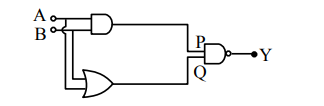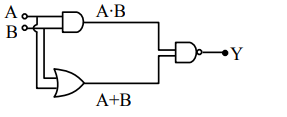# In the following logic circuit the sequenceQuestion:

In the following logic circuit the sequence of the inputs $\mathrm{A}, \mathrm{B}$ are $(0,0),(0,1),(1,0)$ and $(1,1)$. The output $\mathrm{Y}$ for this sequence will be :1. $1,0,1,0$

2. $0,1,0,1$

3. $1,1,1,0$

4. $0,0,1,1$

Correct Option: , 3

Solution:$\mathrm{Y}=\overline{(\mathrm{A} \cdot \mathrm{B}) \cdot(\mathrm{A}+\mathrm{B})}$

$Y)_{(0,0)}=1$

$\mathrm{Y})_{(0,1)}=1$

$\mathbf{Y})_{(1,0)}=1$

$Y)_{(1,1)}=0$

Option (3) is correct